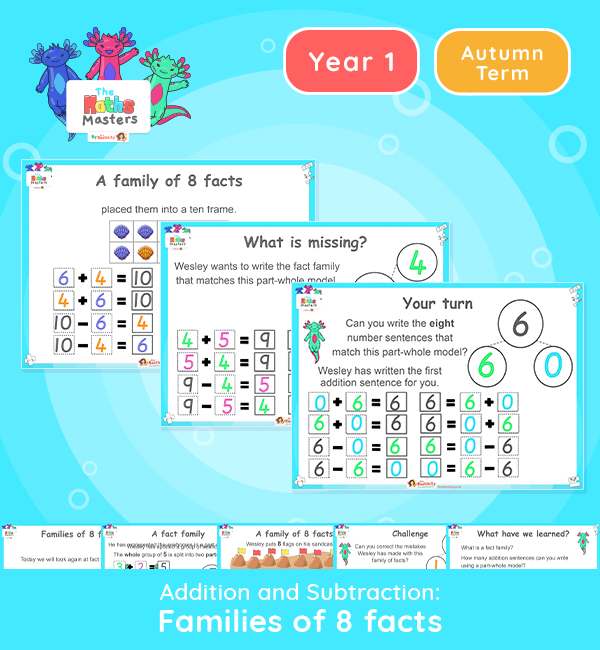# Year 1 | Fact Families: 8 Facts Lesson Presentation## Year 1 addition and subtraction resources

Aligned with the maths mastery approach, this Year 1 | Fact Families: 8 Facts Lesson Presentation is fully editable and designed for the Year 1 maths curriculum to cover the following curriculum objectives:

This lesson focuses on writing families of 8 facts with 4 addition and 4 subtraction sentences.

Small Steps: Fact families  – the 8 facts

NC Links: •Represent and use number bonds and related subtraction facts within 10 •Read, write and interpret mathematical statements involving addition (+), subtraction (-), and equals (=) signs. • Add and subtract one digit numbers to 10, including zero. • Solve one step problems that involve addition and subtraction using concrete objects and pictorial representations.

Ready-to-progress criteria:  1NF-1 Develop fluency in addition and subtraction facts within 10

1AS-2 Read, write and interpret equations continuing addition, subtraction  and equals symbols, and relate additive expressions and equations to real-life contexts.

Previous Experience: Devise and record number stories, using pictures, numbers and symbols.

TAF Statements: Working Towards Add and subtract (one digit numbers) explaining their method verbally in pictures or using apparatus.

Explore our other year 1 addition and subtraction resources.

## Recently Viewed